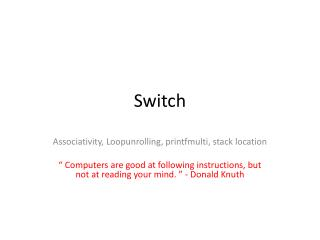DownloadDownload PresentationSwitch

# Switch

Download Presentation## Switch

- - - - - - - - - - - - - - - - - - - - - - - - - - - E N D - - - - - - - - - - - - - - - - - - - - - - - - - - -
##### Presentation Transcript

1. Switch Associativity, Loopunrolling, printfmulti, stack location “ Computers are good at following instructions, but not at reading your mind. ” - Donald Knuth

2. Defect in the switch statement • Perhaps the biggest defect in the switch statement is that cases don't break automatically after the actions for a case label. Once a case statement is executed, the flow of control continues down, executing all the following cases until a break statement is reached. The code switch (2) { case 1: printf("case 1 \n"); case 2: printf("case 2 \n"); case 3: printf("case 3 \n"); case 4: printf("case 4 \n"); default: printf("default \n"); }

3. Print out case 2 case 3 case 4 Default • This is known as "fall through" and was intended to allow common end processing to be done, after some case-specific preparation had occurred. In practice it's a severe misfea-ture, as almost all case actions end with a break;.

4. Multiline messages usingescaped newlines printf( "A favorite children's book \ is 'Thomas the tank engine and the Naughty Enginedriver \ who \ tied down Thomas's boiler safety valve'\n" );

5. Precedence Problems of C Operators

6. Order of Evaluation • The moral of all this is that you should always put parentheses around an expressionthat mixes Booleans, arithmetic, or bit-twiddling with anything else.

7. Excellent advice • Some authorities recommend that there are only two precedence levels to remember in C: multiplication and division come before addition and subtraction. • Everything else should be in parentheses.

8. What "Associativity" Means - Right-associativity of assignment operators What value a will have ? #include <stdio.h> int main(void){ int a, b=1, c=2; a = b = c; printf("Value of A is : %d\n",a); system("pause"); return 0; }

9. Right-associativity of assignment operators • Output: Value of A is : 2 • Every operator has a level of precedence and a "left" or "right" associativity assigned to it. • The Right associativityprotocol says that this means the rightmost operation in the expression is evaluated first • The right-associativity of the = operator allows expressions such as a = b = c to be interpreted as a = (b = c), thereby setting both a and b to the value of c.

10. The stack • The stack provides the storage area for local variables declared inside functions. These are known as "automatic variables" in C terminology.

11. The approximate location of the stackon your system: #include <stdio.h> int main(){ inti; printf("The stack top is near %p\n", &i); system("pause"); return 0; }

12. The standard code optimizations are techniques loop unrolling, in-line function expansion, common subexpression elimination, improved register • allocation, omitting runtime checks on array bounds, loop-invariant code motion, operator strength • reduction (turning exponen-tiation into multiplication, turning multiplication into bit-shiftingand/or addition), and so on.

13. loop unrolling Example Unrolled loop should run faster because of reduction in loop overhead. Normal loop int x; for(x =0; x <100; x++) { printhello(x); }

14. loop unrolling Example • After loop unrolling for (x = 1; x <=100; x+=5) { printhello(x); printhello(x+1); printhello(x+2); printhello(x+3); printhello(x+4); } As a result of this modification, the new program has to make only 20 iterations, instead of 100.

15. Algorithm • Computation is any type of calculation or use of computing technology in information processing.

16. What is the output of the following program? #include<stdio.h> int main() { int a = 5; int b = ++a * a++; printf("%d\n",b); system("pause"); return 0; }

17. What is the output of the following program? • (a) 25 • (b) 30 • (c) 36 • (d) Undefined Behavior

18. What is the output of the following switch program? #include<stdio.h> int main() { int a = 5; switch(a) { default: a = 4; case 6: a--; case 5: a = a+1; case 1: a = a-1; } printf("%d \n",a); system("pause"); return 0; }

19. What is the output of the following switch program? • (a) 5 • (b) 4 • (c) 3 • (d) None of these

20. Quiz – Sum numbers from 1 – to 10 • The general formula is: • (First term + last term) * (number of terms / 2) (1+10)*(10/2) or 55

21. Cyclic nature of signedchar • What will be output if you will compile and execute the following c code? void main(){ char c=125;     c=c+10; printf("%d",c); System(“pause”); }

22. Output • (A) 135 • (B) +INF • (C) -121 • (D) -8 • (E) Compiler error

23. Cyclic nature of signedchar • So, • 125+1= 126 • 125+2= 127 • 125+3=-128 • 125+4=-127 • 125+5=-126 • 125+6=-125 • 125+7=-124 • 125+8=-123 • 125+9=-122 • 125+10=-121

24. Assignment - 3 • Greatest common divisor • The greatest common divisor (gcd) of two or more non-zero integers, is the largest positive integer that divides the numbers without a remainder. For example, the GCD of 8 and 12 is 4.

25. Using Euclid's algorithm To compute gcd(48,18), divide 48 by 18 to get a quotient of 2 and a remainder of 12. Then divide 18 by 12 to get a quotient of 1 and a remainder of 6. Then divide 12 by 6 to get a remainder of 0, which means that 6 is the gcd.